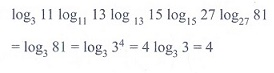# Chapter 2: Basic Algebra - Online Test

Q1. Find a so that the sum and product of the roots of the equation 2x2 + (a - 3)x + 3a - 5 = 0 are equal is
Explaination / Solution: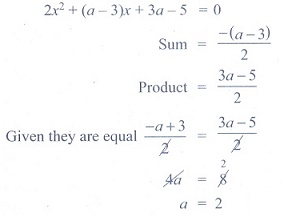Q2.

If a and b are the roots of the equation x2 - kx + 16 = 0 and satisfy a2 + b2 = 32, then the value of k is

Explaination / Solution: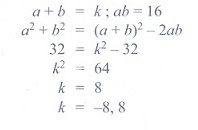Q3. The number of solutions of x2 + |x 1| = 1 is
Explaination / Solution: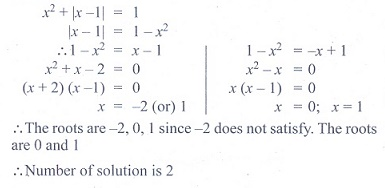Q4. The equation whose roots are numerically equal but opposite in sign to the roots of 3x2 - 5x - 7 = 0 is
Explaination / Solution: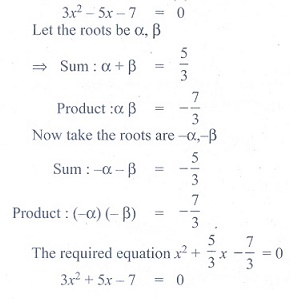Q5.

If 8 and 2 are the roots of x2 +ax+c = 0 and 3, 3 are the roots of x2 +dx+b = 0, then the roots

of the equation x2 + ax + b = 0 are
Explaination / Solution: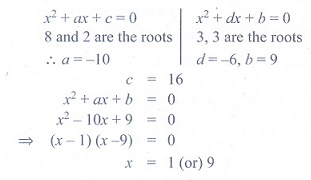Q6.

If a and b are the real roots of the equation x2 - kx + c = 0, then the distance between the points (a, 0) and (b, 0) is

Explaination / Solution: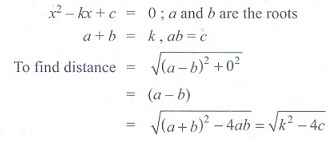Q7. If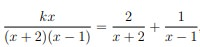, then the value of k is
Explaination / Solution: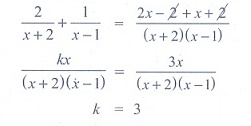Q8. If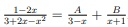, then the value of A + B is
Explaination / Solution: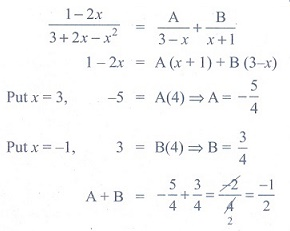Q9. The number of roots of (x + 3)4 + (x + 5)4 = 16 is
Explaination / Solution: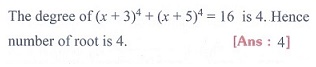Q10. The value of log3 11. log11 13. log13 15.log15 27.log27 81 is
Explaination / Solution: